Anúncio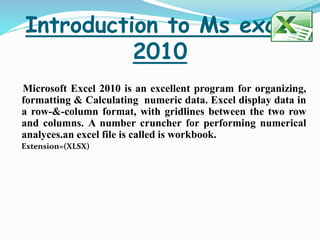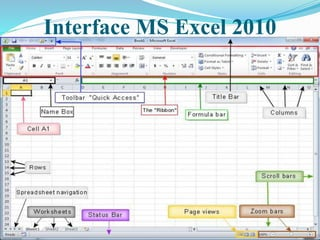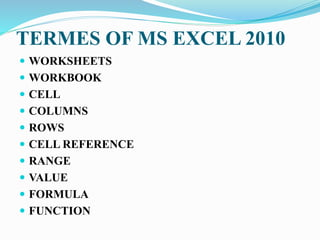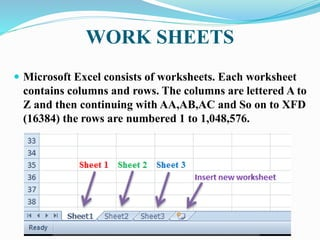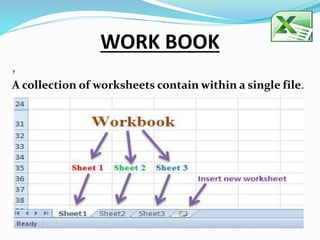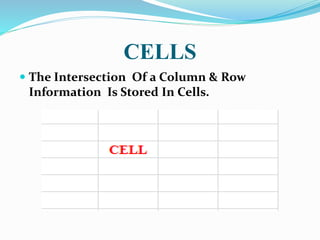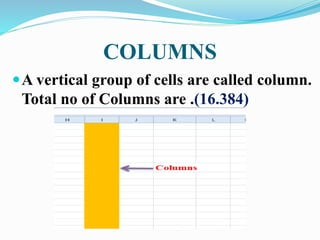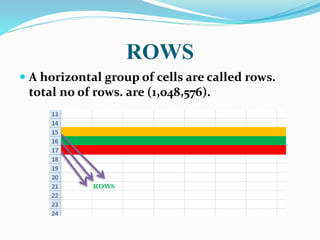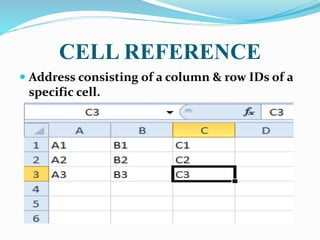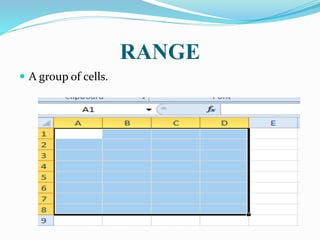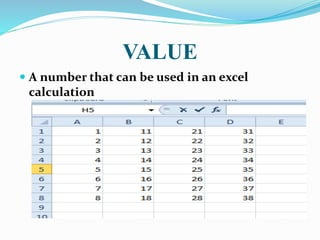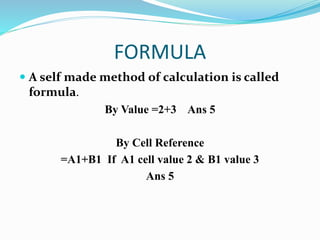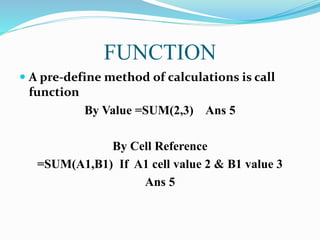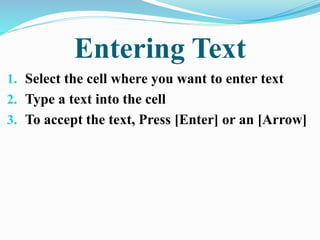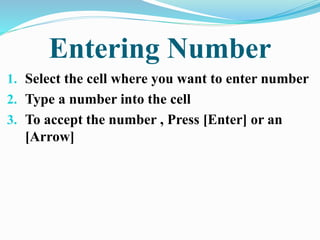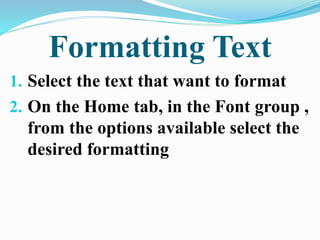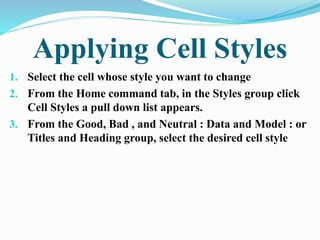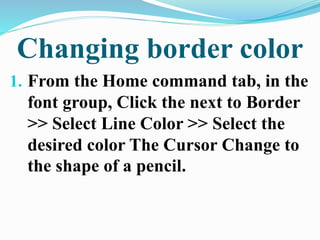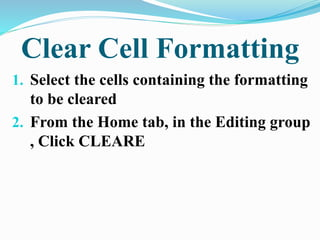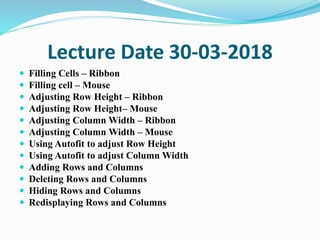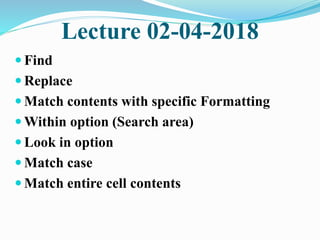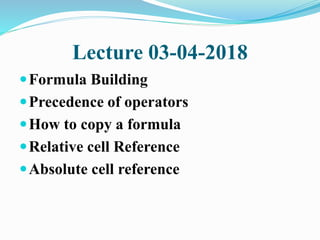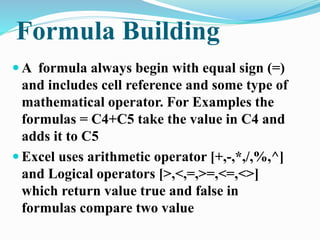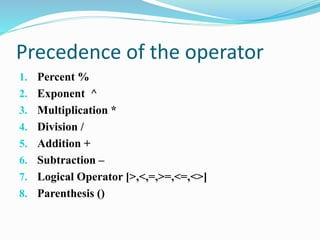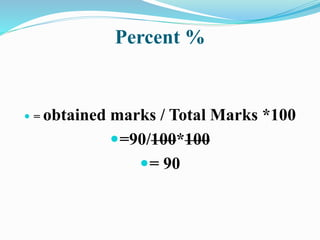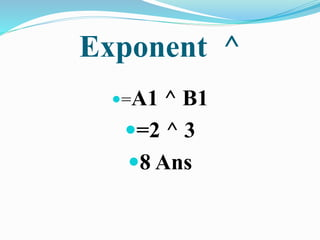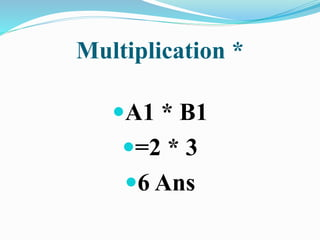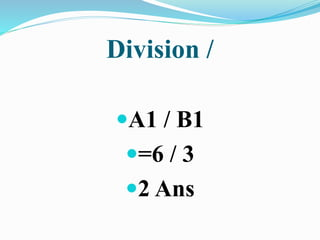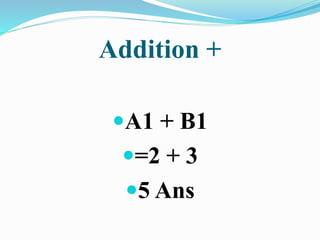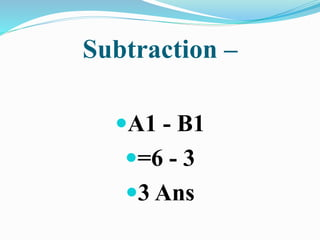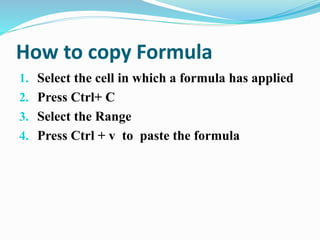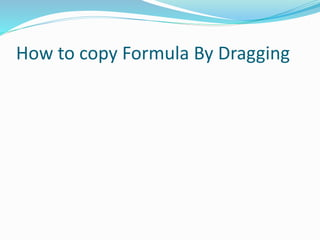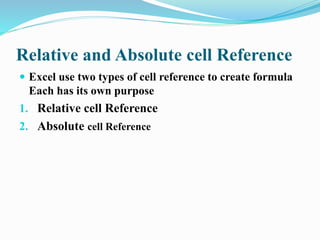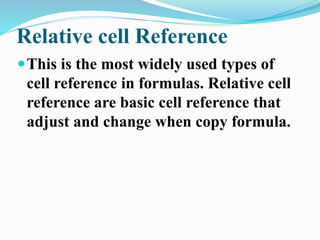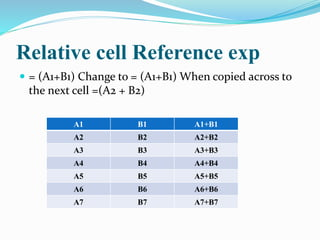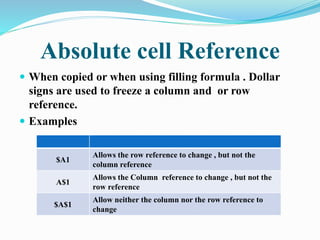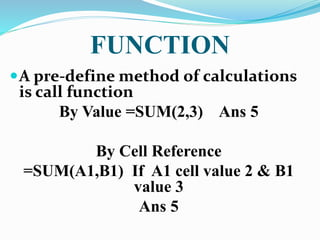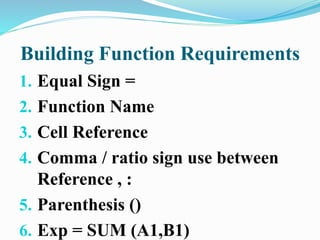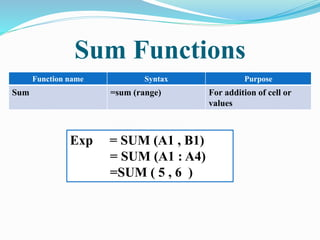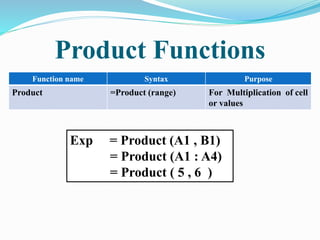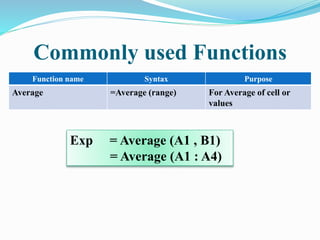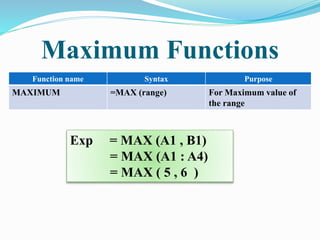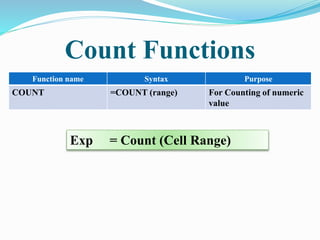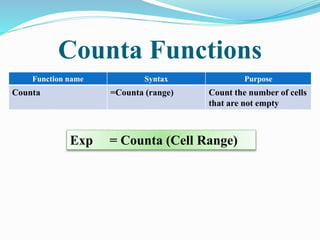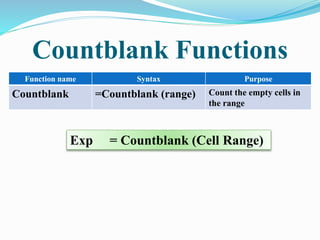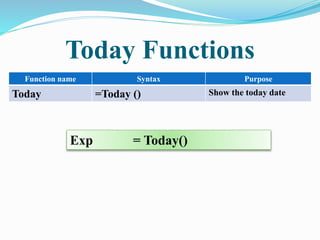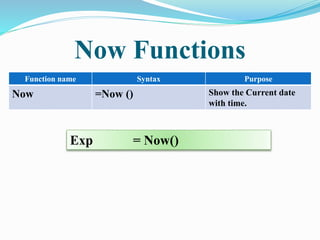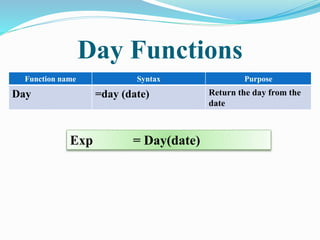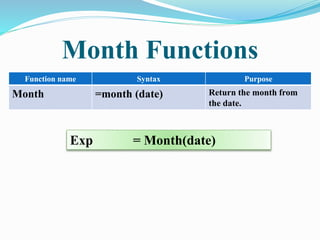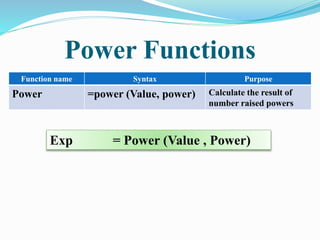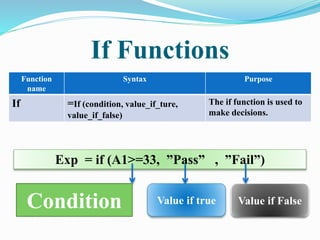1 de 57
Anúncio

### Lesson

1. Today my topic is INTRODUCTION TO EXCEL 2010 & its terms
2. Introduction to Ms excel 2010 Microsoft Excel 2010 is an excellent program for organizing, formatting & Calculating numeric data. Excel display data in a row-&-column format, with gridlines between the two row and columns. A number cruncher for performing numerical analyces.an excel file is called is workbook. Extension=(XLSX)
3. Interface MS Excel 2010
4. TERMES OF MS EXCEL 2010  WORKSHEETS  WORKBOOK  CELL  COLUMNS  ROWS  CELL REFERENCE  RANGE  VALUE  FORMULA  FUNCTION
5. WORK SHEETS  Microsoft Excel consists of worksheets. Each worksheet contains columns and rows. The columns are lettered A to Z and then continuing with AA,AB,AC and So on to XFD (16384) the rows are numbered 1 to 1,048,576.
6. WORK BOOK , A collection of worksheets contain within a single file.
7. CELLS  The Intersection Of a Column & Row Information Is Stored In Cells.
8. COLUMNS A vertical group of cells are called column. Total no of Columns are .(16.384)
9. ROWS  A horizontal group of cells are called rows. total no of rows. are (1,048,576).
10. CELL REFERENCE  Address consisting of a column & row IDs of a specific cell.
11. RANGE  A group of cells.
12. VALUE  A number that can be used in an excel calculation
13. FORMULA  A self made method of calculation is called formula. By Value =2+3 Ans 5 By Cell Reference =A1+B1 If A1 cell value 2 & B1 value 3 Ans 5
14. FUNCTION  A pre-define method of calculations is call function By Value =SUM(2,3) Ans 5 By Cell Reference =SUM(A1,B1) If A1 cell value 2 & B1 value 3 Ans 5
15. Entering Text 1. Select the cell where you want to enter text 2. Type a text into the cell 3. To accept the text, Press [Enter] or an [Arrow]
16. Entering Number 1. Select the cell where you want to enter number 2. Type a number into the cell 3. To accept the number , Press [Enter] or an [Arrow]
17. Today Lecture 28-03-2018 1. Formatting Text 2. Applying Cell Styles 3. Changing Borders 4. Changing border color 5. Creating Cell Merge 6. Removing Cell Merge 7. Wrapping Text 8. Clear Cell Formatting
18. Formatting Text 1. Select the text that want to format 2. On the Home tab, in the Font group , from the options available select the desired formatting
19. Applying Cell Styles 1. Select the cell whose style you want to change 2. From the Home command tab, in the Styles group click Cell Styles a pull down list appears. 3. From the Good, Bad , and Neutral : Data and Model : or Titles and Heading group, select the desired cell style
20. Changing Borders 1. Select the cell Whose borders you want to format 2. From the Home command tab, in the font group, Click the next to Border & Select the desired border .
21. Changing border color 1. From the Home command tab, in the font group, Click the next to Border >> Select Line Color >> Select the desired color The Cursor Change to the shape of a pencil.
22. Creating Cell Merge 1. Select the cell you want to merge 2. From the Home command tab, in the Alignment group, Click Merge & Center the cells are merged and the text aligns to the center
23. Removing Cell Merge 1. Select the cell you want to unmerge 2. Click the > next to Merge & Center >> Select the Unmerge cell
24. Wrapping Text 1.Select the appropriate cells 2.From the Home Command tab, in the Alignment group, Click Wrapping text
25. Clear Cell Formatting 1. Select the cells containing the formatting to be cleared 2. From the Home tab, in the Editing group , Click CLEARE
26. Lecture Date 30-03-2018  Filling Cells – Ribbon  Filling cell – Mouse  Adjusting Row Height – Ribbon  Adjusting Row Height– Mouse  Adjusting Column Width – Ribbon  Adjusting Column Width – Mouse  Using Autofit to adjust Row Height  Using Autofit to adjust Column Width  Adding Rows and Columns  Deleting Rows and Columns  Hiding Rows and Columns  Redisplaying Rows and Columns
27. Lecture 02-04-2018  Find  Replace  Match contents with specific Formatting  Within option (Search area)  Look in option  Match case  Match entire cell contents
28. Lecture 03-04-2018 Formula Building Precedence of operators How to copy a formula Relative cell Reference Absolute cell reference
29. Formula Building  A formula always begin with equal sign (=) and includes cell reference and some type of mathematical operator. For Examples the formulas = C4+C5 take the value in C4 and adds it to C5  Excel uses arithmetic operator [+,-,*,/,%,^] and Logical operators [>,<,=,>=,<=,<>] which return value true and false in formulas compare two value
30. Precedence of the operator 1. Percent % 2. Exponent ^ 3. Multiplication * 4. Division / 5. Addition + 6. Subtraction – 7. Logical Operator [>,<,=,>=,<=,<>] 8. Parenthesis ()
31. Percent %  = obtained marks / Total Marks *100 =90/100*100 = 90
32. Exponent ^ =A1 ^ B1 =2 ^ 3 8 Ans
33. Multiplication * A1 * B1 =2 * 3 6 Ans
34. Division / A1 / B1 =6 / 3 2 Ans
35. Addition + A1 + B1 =2 + 3 5 Ans
36. Subtraction – A1 - B1 =6 - 3 3 Ans
37. How to copy Formula 1. Select the cell in which a formula has applied 2. Press Ctrl+ C 3. Select the Range 4. Press Ctrl + v to paste the formula
38. How to copy Formula By Dragging
39. Relative and Absolute cell Reference  Excel use two types of cell reference to create formula Each has its own purpose 1. Relative cell Reference 2. Absolute cell Reference
40. Relative cell Reference This is the most widely used types of cell reference in formulas. Relative cell reference are basic cell reference that adjust and change when copy formula.
41. Relative cell Reference exp  = (A1+B1) Change to = (A1+B1) When copied across to the next cell =(A2 + B2) A1 B1 A1+B1 A2 B2 A2+B2 A3 B3 A3+B3 A4 B4 A4+B4 A5 B5 A5+B5 A6 B6 A6+B6 A7 B7 A7+B7
42. Absolute cell Reference  When copied or when using filling formula . Dollar signs are used to freeze a column and or row reference.  Examples \$A1 Allows the row reference to change , but not the column reference A\$1 Allows the Column reference to change , but not the row reference \$A\$1 Allow neither the column nor the row reference to change
43. FUNCTION A pre-define method of calculations is call function By Value =SUM(2,3) Ans 5 By Cell Reference =SUM(A1,B1) If A1 cell value 2 & B1 value 3 Ans 5
44. Building Function Requirements 1. Equal Sign = 2. Function Name 3. Cell Reference 4. Comma / ratio sign use between Reference , : 5. Parenthesis () 6. Exp = SUM (A1,B1)
45. Sum Functions Function name Syntax Purpose Sum =sum (range) For addition of cell or values Exp = SUM (A1 , B1) = SUM (A1 : A4) =SUM ( 5 , 6 )
46. Product Functions Function name Syntax Purpose Product =Product (range) For Multiplication of cell or values Exp = Product (A1 , B1) = Product (A1 : A4) = Product ( 5 , 6 )
47. Commonly used Functions Function name Syntax Purpose Average =Average (range) For Average of cell or values Exp = Average (A1 , B1) = Average (A1 : A4)
48. Maximum Functions Function name Syntax Purpose MAXIMUM =MAX (range) For Maximum value of the range Exp = MAX (A1 , B1) = MAX (A1 : A4) = MAX ( 5 , 6 )
49. Count Functions Function name Syntax Purpose COUNT =COUNT (range) For Counting of numeric value Exp = Count (Cell Range)
50. Counta Functions Function name Syntax Purpose Counta =Counta (range) Count the number of cells that are not empty Exp = Counta (Cell Range)
51. Countblank Functions Function name Syntax Purpose Countblank =Countblank (range) Count the empty cells in the range Exp = Countblank (Cell Range)
52. Today Functions Function name Syntax Purpose Today =Today () Show the today date Exp = Today()
53. Now Functions Function name Syntax Purpose Now =Now () Show the Current date with time. Exp = Now()
54. Day Functions Function name Syntax Purpose Day =day (date) Return the day from the date Exp = Day(date)
55. Month Functions Function name Syntax Purpose Month =month (date) Return the month from the date. Exp = Month(date)
56. Power Functions Function name Syntax Purpose Power =power (Value, power) Calculate the result of number raised powers Exp = Power (Value , Power)
57. If Functions Function name Syntax Purpose If =If (condition, value_if_ture, value_if_false) The if function is used to make decisions. Exp = if (A1>=33, ”Pass” , ”Fail”) Condition Value if true Value if False
Anúncio